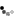Loading... Please wait...

# How much does a gas fire cost to run?

Posted

In essence this is quite a simple question, you simply multiply the cost of your gas per KW/h by the input on the gas fire and this will give you the cost per hour to run.

On all of our products you will see a data table, which contains the specification of your chosen fire. Part of this table is the gas input, this is the figure you need to work out the cost of running the fire.

Then have a look at your gas bill and find the cost per unit (KW/h).

If you multiply your unit cost by the input you will get the cost to run the fire per hour.

So if a fire has a 6.9KW input and your gas costs 5p per KW/h then it would cost 34.5p per hour to run the fire.

Please note the cost per unit of gas can vary massively and so it is important to check your own bill for the cost per KW/h.